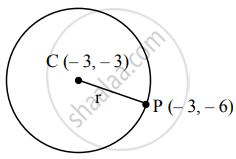# Find the equation of the circle with centre at (−3, −3) passing through the point (−3, −6) - Mathematics and Statistics

Sum

Find the equation of the circle with centre at (−3, −3) passing through the point (−3, −6)

#### SolutionCentre of the circle is C (– 3, – 3) and it passes through the point P (– 3, – 6).

By distance formula

Radius (r) = CP = sqrt([-3 - (-3)]^2 + [- 6 - (- 3)]^2

= sqrt((-3 + 3)^2 + (-6 + 3)^2

= sqrt(0^2 + (-3)^2

= sqrt(9)

= 3

The equation of a circle with centre at (h, k) and radius ‘r’ is given by

(x – h)2 + (y – k)2 = r2

Here, h = – 3, k = –3, r = 3

∴ The required equation of the circle is

[x – (– 3)]2 + [y – (– 3)]2 = 32

∴ (x + 3)2 + (y + 3)2 = 9

∴ x2 + 6x + 9 + y2 + 6y + 9 – 9 = 0

∴ x2 + y2 + 6x + 6y + 9 = 0.

Is there an error in this question or solution?